# 3.2 Theoretical background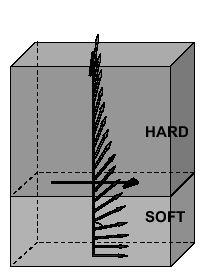The reduction of the coercivity in a soft/hard magnetic bilayer is explained by the formation of an exchange spring: the reversal starts in the soft phase (FeRh in our case), then a domain wall is formed, which later penetrates into the hard phase (FePt) and helps to reverse its magnetization. This mechanism has also received recently the name of domain wall assisted magnetic recording (DWAMR) [Dobin 06]. In this case the size of the soft phase should be larger than the domain wall widthwhereis the micromagnetic exchange constant in the soft layer and- the saturation magnetization. Alternatively, R. Victora and X. Shen [Victora 05a] have proposed a mechanism in which the domain wall is not formed, and the two layers behave essentially as two-spins: initially, the soft layer rotates towards the external field, which exerts an additional torque to the hard layer, helping to invert its magnetization. Both mechanisms are schematically represented in Fig. 3.5.The standard approach to describe conventional in-plane exchange springs
[Kneller 91,Hernando 92] used in many previous publications and generalized recently to the case of the perpendicular exchange spring [Guslienko 04] is a 1D model. Essentially, it assumes the homogeneous in-plane magnetization and considers a set of atomistic planes with the properties of soft or hard phase, each one represented by one magnetic moment. The magnetostatic contribution is taken into account in the demagnetization field approximation, which considers the in-plane dimensions as infinite. The model admits analytical treatment.

In the two macrospins model the coercive field is determined by an average anisotropy field [Fullerton 98]:(3.1)

where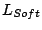is the thickness of the soft layer,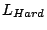is the thickness of the hard layer,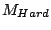the saturation magnetization of the hard layer,is the anisotropy constant of the soft layer and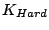is the anisotropy constant of the hard layer. In the same model the corresponding energy barrier is: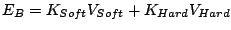(3.2)

whereis the volume of the soft layer,is the volume of the hard layer. These formulae are valid if the thicknesses of both layers are below their respective domain wall widths.

For the exchange spring mechanism the magnetization process is determined by nucleation and depinning field. It has been shown [Goto 65], for example, that the nucleation field is determined in the strongly coupled case by:(3.3)

From this equation, it is clear that the nucleation field increases with the thickness of the soft layer. Namely, whenwe have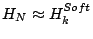.

When the pinning is effective and the interfacial exchange strong enough the coercive field is determined by the pinning field [Kronmüller 02]:(3.4)

whereis the exchange constant in the hard layer. An interesting consequence of the pinning field equation is that the reduction of coercivity is larger when the difference of anisotropy constants between soft and hard materials is smaller. This fact has stimulated later the proposal of the graded media [Suess 06], using a multilayer with increasing anisotropy constant in each layer. In this case large reduction of the coercive field together with large thermal stability have been predicted.

When the interfacial exchange is weak the soft layer nucleates but the domain wall is very sharp. For this case K. Guslienko has derived an expression for the coercive field [Guslienko 04]: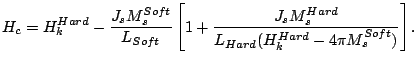(3.5)

Although the equation is not valid for all interfacial exchange, it shows that the coercivity reduces with increasing interfacial exchange parameter. The coercivity will saturate for strong interfacial coupling and depending on the system parameters the behavior will reach that of an exchange spring mechanism (3.4) or two-macrospins (3.3).

The analytical and 1D numerical calculations from K. Guslienko et al.
[Guslienko 04] have shown that the coercivity reduction in the FePt layer due to the exchange coupling to the FeRh layer strongly depends on the interfacial exchange parameter and even for the exchange parameter as low as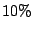of the bulk value, a coercivity reduction of the FePt of up to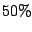could be expected.

However, although a 1D approach is probably justified in bilayers with a good in-plane film quality, the exact reversal mechanism and the degree of interfacial energy required for maximum coercivity reduction in a soft/hard magnetic material are questions requiring a 3D calculation [Suess 05a,Garcia-Sanchez 06]. With 3D simulations, which correctly include the magnetostatic fields, we can study the finite size effects and magnetization inhomogeneities, allowing noncoherent reversal, and variation of the parameters inside the plane, as for example granular structure.

2008-04-04